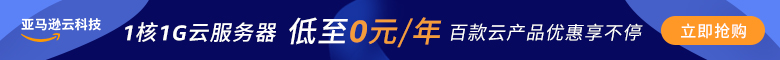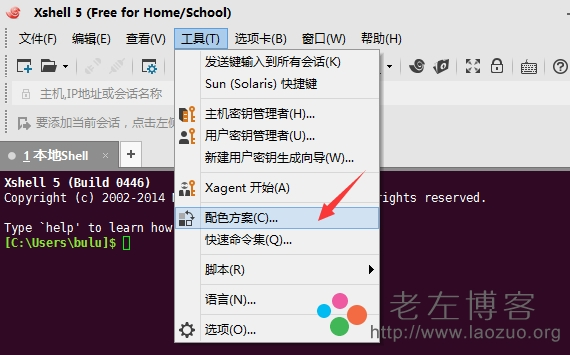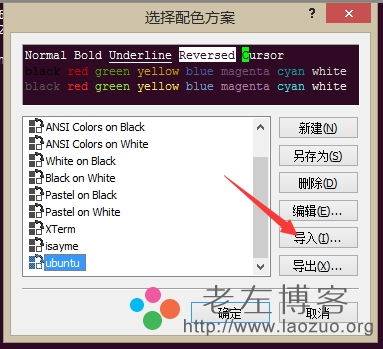# 分享2款XSHELL配色方案及导入配色方案的方法```[isayme]
text(bold)=eaeaea
magenta(bold)=ff00ff
text=ffffff
white(bold)=eaeaea
green=00c000
red(bold)=d20000
green(bold)=00ff00
black(bold)=808080
red=c00000
blue=113fcc
black=000000
blue(bold)=0080ff
yellow(bold)=ffff00
cyan(bold)=00ffff
yellow=c0c000
magenta=c000c0
background=222222
white=c0c0c0
cyan=00c0c0
[Names]
count=1
name0=isayme```

```[ubuntu]
text(bold)=ffffff
text=ffffff
white(bold)=eeeeec
green=4e9a06
red(bold)=ef2929
green(bold)=8ae234
black(bold)=555753
red=cc0000
blue=3465a4
black=000000
blue(bold)=729fcf
yellow(bold)=fce94f
cyan(bold)=34e2e2
yellow=c4a000
magenta=75507b
background=300a24
white=d3d7cf
cyan=06989a
[Names]
count=1
name0=ubuntu```

### XSHELL配色方案的导入方法

A -工具-颜色方案B -导入xshell color scheme配色方案